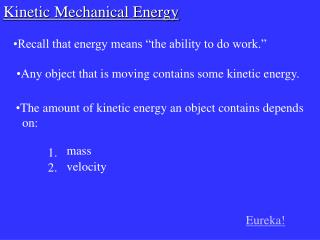DownloadDownload PresentationKinetic Mechanical Energy

# Kinetic Mechanical Energy

Download Presentation## Kinetic Mechanical Energy

- - - - - - - - - - - - - - - - - - - - - - - - - - - E N D - - - - - - - - - - - - - - - - - - - - - - - - - - -
##### Presentation Transcript

1. Kinetic Mechanical Energy • Recall that energy means “the ability to do work.” • Any object that is moving contains some kinetic energy. • The amount of kinetic energy an object contains depends • on: • 1. • 2. mass velocity Eureka!

2. where, EK = kinetic energy, (J) m = mass, (kg) v = velocity, (m/s)

3. Example #1 How fast would a 6.0 kg curling stone be travelling if it contained 48 J of kinetic energy?

4. where, Eg = gravitational potential energy, (J) m = mass, (kg) g = 9.81 N/kg h = height, (m) Gravitational Potential Energy • Gravitational Potential Energy depends on the height an • object is raised above the ground, as well as its mass. Example #2 Find the work done in lifting a 75 kg rock 4.0 m above the ground.

5. Example #3 If an 150 kg mountain lion has 2400 J of energy, how high above the ground is it? Eureka!

6. Homework: pg. 136 #1-9